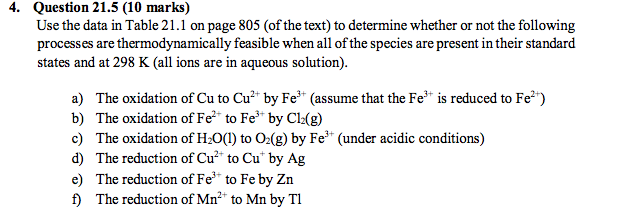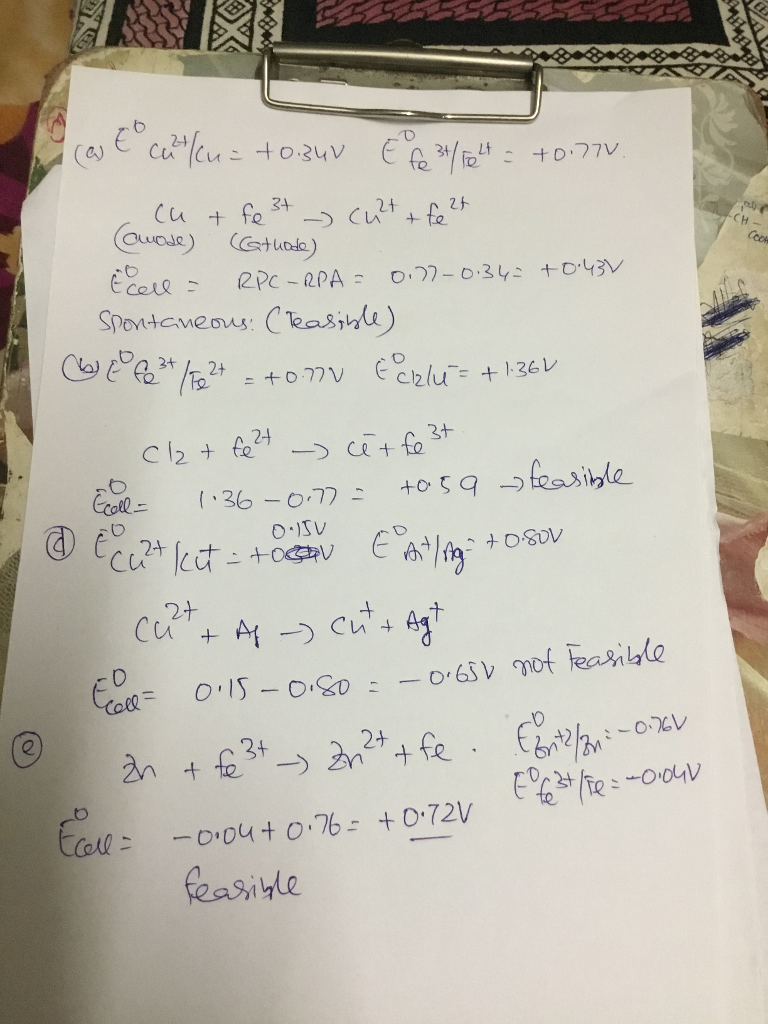# Homework Solution: Use the data in Table 21.1 on page 805 (of the text) to determine whether or not the following…Use the data in Table 21.1 on page 805 (of the text) to determine whether or not the following processes are thermodynamically feasible when all of the species are present in their standard states and at 298 K (all ions are in aqueous solution). a) The oxidation of Cu to Cu^2+ by Fe^3+ (assume that the Fe^3+ is reduced to Fe^2+) b) The oxidation of Fe^2+ to Fe^3+ by Cl_2(g) c) The oxidation of H_2O(1) to O_2(g) by Fe^3+ (under acidic conditions) d) The reduction of Cu^2+ to Cu^+ by Ag e) The reduction of Fe^3+ to Fe by Zn f) The reduction of Mn^2+ to Mn by T1